본문바로가기

## 검색결과

검색결과 (전체 1건)

### 검색결과제한

열기
• 소장도서관
• 원문
• 발행년도
-
• 언어
• 원문이용안내
•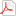모든 이용자
•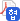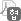협정기관 이용자
•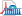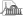국회도서관 방문 이용자
• 원문아이콘이 없는 자료는 국회도서관에 방문하여 책자로만 이용이 가능합니다.

논문명/저자명
Casting defect-affected fatigue life modeling for cast aluminum alloys / 장영환
발행사항
진주 : 경상대학교 대학원, 2008.8
청구기호
TD 669 -8-96
형태사항
xi, 142 p. ; 26 cm
자료실
전자자료
제어번호
KDMT1200871963
주기사항
학위논문(박사) -- 경상대학교 대학원, Metallurgical and Materials Engineering, 2008.8
원문

목차보기더보기

title page

Contents

초록 12

I. Introduction 15

1. Background and objectives 15

2. Approaches 21

II. Theoretical background and literature review 25

1. General aspects of crack initiation 25

2. Linear elastic fracture mechanics (LEFM) 27

3. Fatigue crack propagation (FCP) 29

4. Fatigue behavior of castings 32

1) Crack initiation 32

2) FCP behavior in cast aluminum 33

3) Fatigue life modeling of cast aluminum alloy 34

III. Experimental procedures 43

1. Material 43

2. Microstructure examination 46

3. Mechanical properties 46

4. S-N fatigue test 47

5. Staircase fatigue test 48

6. Fatigue crack propagation (FCP) test 49

IV. Results and discussion 58

1. Microstructure 58

2. S-N fatigue property of cast A319-T7 aluminum alloy 66

3. Fractographic analysis 70

1) Crack initiation sites from room temperature tested specimens 70

2) Crack initiation sites from elevated temperature tested specimens 71

3) Fatigue crack initiation mechanism in defect-free specimen at elevated temperature 74

4) Characteristics for fatigue-fractured specimens 76

5) Weibull statistical analysis for initial flaw size[내용누락;p.96] 80

4. FCP behavior in cast A319-T7 aluminum 111

5. Fatigue life prediction in cast A319-T7 aluminum alloy 118

1) Approaches to fatigue life modeling 118

2) Fatigue life prediction in cast A319-T7 aluminum alloy based on LEFM principles 121

6. Fatigue life prediction in die-cast AM60B magnesium alloy 137

7. Comparison of predicted fatigue life using LEFM model and small crack model 144

V. Conclusions 150

References 152

감사의 글 157

Table 3.1. Chemical composition of A319 aluminum alloy used in this study 44

Table 3.2. Mechanical properties of cast A319-T7 aluminum alloy at room temperature 53

Table 4.1. Microstructural features observed from metallographic samples 65

Table 4.2. Fatigue strength of cast A319-T7 aluminum alloy 69

Table 4.3. Summary of the characteristics for fatigue-fractured specimens at room temperature 98

Table 4.4. Summary of the characteristics for fatigue-fractured specimens at 150°C 100

Table 4.5. Effect of flaw geometry on the fatigue life of cast A319-T7 aluminum alloy with an initial center semi-circular surface flaw, showing the predicted fatigue life at a maximum applied stress of 100 and 160 MPa(R=-1) 108

Table 4.6. Predicted Weibull statistical parameters for EMS and depth of initial crack measured from fatigue crack initiators in cast A319-T7 aluminum alloy[내용누락;p.96] 110

Table 4.7. ΔKth, Paris constant (C) and exponent (m), and KQ determined from dα/dN-ΔK curves(이미지참조) 114

Table 4.8. AFGROW input parameters for cast A319-T7 aluminum alloy 127

Table 4.9. AFGROW input parameters (ΔKeff) for cast A319-T7 aluminum alloy(이미지참조) 134

Table 4.10. Summary of the characteristics for fatigue-fractured die-cast AM60B magnesium specimens at an R ratio of -1 141

Table 4.11. AFGROW input parameters for die-cast AM60B magnesium alloy 142

Figure 1.1. S-N curves with different porosity for cast 295 aluminum alloy. 18

Figure 1.2. Typical SEM fractograph of cast A319-T7 aluminum alloy. 19

Figure 1.3. Definition of defect area suggested by Murakami et al. 20

Figure 1.4. Schematic illustration for total fatigue life, Nf, of (a) Nf = Ni + Np and (b) Nf = Np (for castings), respectively.(이미지참조) 23

Figure 1.5. Schematic illustration of fracture mechanics approaches to predict fatigue life. 24

Figure 2.1. Schematic illustration of crack initiation in atomic scale. 26

Figure 2.2. Schematic illustration of typical stress/crack geometries and corresponding stress intensity factor, K, in cracked component. 28

Figure 2.3. Schematic plot of dα/dN-ΔK curve on a log-log scale. 31

Figure 2.4. Micrograph of Sr-modified 319 lost-foam casting with both gas- and shrinkage-driven pores. 37

Figure 2.5. Fatigue crack initiated from slip bands in hot isostatic pressed A356 alloy. 38

Figure 2.6. Schematic illustration of the behavior of small fatigue cracks in comparison to long cracks. 39

Figure 2.7. Schematic illustration of general crack closure mechanisms 40

Figure 2.8. Predicted fatigue life of cast CP601 aluminum alloy based on LEFM model for tests at R ratio of -1. 41

Figure 2.9. Predicted fatigue life of cast W319-T7 aluminum alloy based on small crack model for tests at R ratio of -1. 42

Figure 3.1. As-fabricated engine block. 45

Figure 3.2. Specimen dimensions (unit : mm) used for (a) tensile test and (b) shear test, respectively. 51

Figure 3.3. (a) Stress-strain and (b) shear stress-displacement curves of cast A319-T7 aluminum alloy at room temperature, respectively. 52

Figure 3.4. Specimen dimensions (unit : mm) used for the S-N fatigue test. 54

Figure 3.5. S-N fatigue testing system with a griped specimen. 55

Figure 3.6. Schematic diagrams (unit: mm) of compact tension (CT) specimen used for the FCP test. 56

Figure 3.7. (a) Schematic illustration of FCP test using the DCPD method and (b) a wired specimen. 57

Figure 4.1. (a) As-fabricated engine block used in this study and (b) as-cut sample from bulkhead (white square in Figure 4.1(a)), respectively, and optical micrographs of the (c) area A and (d) area B, as marked in Figure 4.1(b). 60

Figure 4.2. Optical macrograph showing the grains and highlighted grain boundaries of cast A319-T7 aluminum alloy. 61

Figure 4.3. Optical micrographs of (a) low- and (b) high-magnification for cast A319-T7 aluminum alloy observed in area A of Figure 4.1(b). 62

Figure 4.4. SEM micrograph showing the detail of casting defects. 63

Figure 4.5. EIFS distributions in cast A319-T7 aluminum alloy observed from the metallographic samples. 64

Figure 4.6. (a) S-N fatigue data for cast A319-T7 aluminum alloy at 25℃ and 150℃, and (b) staircase test results at 150℃, respectively. 68

Figure 4.7. (a) Low- and (b) high-magnification (detail of region I, showing the pores responsible for crack initiation) SEM fractographs of fatigue-fractured specimen at room temperature (σmax = 110 MPa, Nf = 534,143 cycles, R = -1)....(이미지참조) 83

Figure 4.8. (a) Low- and (b) high-magnification (detail of crack initiation site) SEM fractographs of fatigue-fractured specimen at room temperature (σmax = 100 MPa, Nf= 1,568,513 cycles, R = -1)(이미지참조) 84

Figure 4.9. SEM fractographs of (a) pore (Nf = 413,495 cycles) and (b) oxide film (Nf = 1,894,836 cycles) on crack initiation site at 25°C under σmax = 100 MPa.(이미지참조) 85

Figure 4.10. (a), (b) and (c) matching EDS results taken in the area I, II and III, respectively, as marked in Figure 4.9(b). 86

Figure 4.11. SEM fractographs of fatigue-fractured specimens under (a) σmax = 74 MPa (Nf = 5,662,027 cycles), (b) σmax = 77.5 MPa (Nf = 2,083,543 cycles), (c) σmax = 77.5 MPa (Nf= 7,218,002 cycles), (d) σmax = 77.5 MPa (Nf = 8,679,370...(이미지참조) 87

Figure 4.12. (a) Detail of the area A as marked in Figure 4.11(c), and (b) and (c) the matching EDS results in the area I and II, respectively, as marked in Figure 4.12(a). 89

Figure 4.13. (a) Detail of the area B, as marked in Figure 4.11(g), and (b), (c) and (d) the matching EDS results in the area III, IV and VI, respectively, as marked in Figure 4.13(a). 90

Figure 4.14. S-N curve of cast A319-T7 aluminum alloy fatigue-tested at 150℃, showing the correlation between fatigue crack initiators and fatigue life. 91

Figure 4.15. (a) Low-magnification SEM side-view of the specimen (σmax = 74 MPa, 150°C, Nf = 5,662,027 cycles) as shown in Figure 4.11(a), and (b) detail of the area A, showing the slip band that formed on the specimen surface.(이미지참조) 92

Figure 4.16. (a) Low- and (b) high-magnification SEM fractographs of tensile-fractured specimen after runout under σmax = 74 MPa at 150°C, showing the crack formed during fatigue test. (c) Enlargement of the area A in the Figure 4.16(b),...(이미지참조) 93

Figure 4.17. SEM fractograph of tensile-fractured specimen after runout under σmax = 77.5 MPa at 150°C, showing that pores exist at the subsurface.(이미지참조) 94

Figure 4.18. Schematic illustrations of crack initiation mechanism caused by slip band in cast Al alloy. 95

Figure 4.19. Schematic illustrations for crack initiation mechanism at environment-induced slip plane: (a) exposure of fresh metal susrface; (b) absorption of oxygen; (c) crack initiation caused by local decohesion. 96

Figure 4.20. Low-magnification SEM fractograph of fatigue-fractured specimen at room temperature (σmax = 85 MPa, Nf = 712,371 cycles, R = -1), showing the depth (a) and width (2c) of initial crack for failure-dominant defect.(이미지참조) 97

Figure 4.21. EIFS distributions calculated from the projected area of failure-dominant defects. 102

Figure 4.22. (a) Correlation of fatigue test results with EIFS and (b) change in fatigue life as a function of EIFS at same stress, respectively. 103

Figure 4.23. Change in geometry factor, f(g), as a function of initial α/D (Eq. 4-2). 104

Figure 4.24. Correlation of fatigue life with (a) initial K√A,max by using the √area parameter, and (b) initial Ka,max by using the depth of initial crack for failure-dominant defects at room temperature, respectively.(이미지참조) 105

Figure 4.25. Correlation of fatigue life with (a) initial K√A,max by using the √area parameter, and (b) initial Ka,max by using the depth of initial crack for failure-dominant defects at 150°C, respectively.(이미지참조) 106

Figure 4.26. Schematic illustrations of surface flaw geometry. 107

Figure 4.27. Two parameter Weibull plot for EIFS and depth of initial crack measured from fatigue crack initiators in cast A319-T7 aluminum alloy. 109

Figure 4.28. (a) dα/dN-ΔK curves for cast A319-T7 aluminum alloy at temperatures of 25°C and 150°C, and (b) the Paris's equation (dα/dN = C(ΔK)m), respectively.(이미지참조) 113

Figure 4.29. Optical micrographs of fatigue crack path at 25°C for cast A319-T7 aluminum alloy at (a) ΔK = 6 MPa√m, (b) ΔK = 8 MPa√m and (c) ΔK = 15 MPa√m, respectively. The FCP direction was from left to right.(이미지참조) 115

Figure 4.30. Optical micrographs of fatigue crack path at 150°C for cast A319-T7 aluminum alloy at (a) ΔK = 4.5 MPa√m, (b) ΔK = 8 MPa√m and (c) ΔK = 15 MPa√m, respectively. The FCP direction was from left to right.(이미지참조) 116

Figure 4.31. Schematic illustration of FCP behavior at temperatures of 25℃ and 150℃. 117

Figure 4.32. (a) Overall fatigue fracture surface and (b) the crack configuration used in the rod-standard solution, respectively. 128

Figure 4.33. (a) Experimental fatigue data and predicted S-N curves for cast A319-T7 aluminum alloy, indicting that the predicted fatigue life with different αi was calculated based on conventional LEFM-ΔK model. (b) Comparison of the...(이미지참조) 129

Figure 4.34. Schematic illustration of back-calculated EIFS, αi,corr, to predict conservative life.(이미지참조) 130

Figure 4.35. Relationships between EIFS (αi) measured from fractographs and back-calculated EIFS (αi,corr) at (a) 25°C and (b) 150°C, respectively.(이미지참조) 131

Figure 4.36. Experimental fatigue data and predicted S-N curves for cast A319-T7 aluminum alloy at (a) 25°C and (b) 150°C, respectively, based on modified LEFM-ΔK model. 132

Figure 4.37. Experimental fatigue data and predicted S-N curves with different am values for cast A319-T7 aluminum alloy at (a) 25°C and (b) 150°C, respectively, based on modified LEFM -ΔK model.(이미지참조) 133

Figure 4.38. Relationship between EIFS (αi) and back-calculated EIFS (αi,corr) using LEFM-ΔKeff.(이미지참조) 135

Figure 4.39. Experimental fatigue data and predicted S-N curves for cast A319-T7 aluminum alloy at room temperature based on modified LEFM-ΔKeff model.(이미지참조) 136

Figure 4.40. Fatigue property for die-cast AM60B magnesium alloy at an R ratio of -1, indicating the crack initiation site. 139

Figure 4.41. Fatigue life of die-cast AM60B magnesium alloy as a function of maximum stress intensity factor, Kmax, at an R ratio of -1, assuming pores at crack initiation site as initial cracks.(이미지참조) 140

Figure 4.42. Experimental fatigue data and predicted S-N curve for die-cast AM60B magnesium alloy at an R ratio of -1, based on modified LEFM-ΔK model. 143

Figure 4.43. Experimental fatigue data  and predicted S-N curves for cast W319-T7 aluminum alloy with low SDAS of 23 ㎛ at an R ratio of - 1, based on modified LEFM-ΔK model. 147

Figure 4.44. Comparison of predicted fatigue life using LEFM model, modified LEFM-ΔK model and small crack model, showing that the experimental data and predicted fatigue life with the exception of modified LEFM-ΔK model reported by... 148

Figure 4.45. Relationship between EIFS, αi, and magnification factor, αm, in several cast materials.(이미지참조) 149

## 권호기사보기

권호기사 목록 테이블로 기사명, 저자명, 페이지, 원문, 기사목차 순으로 되어있습니다.
기사명 저자명 페이지 원문 기사목차
연속간행물 팝업 열기 연속간행물 팝업 열기